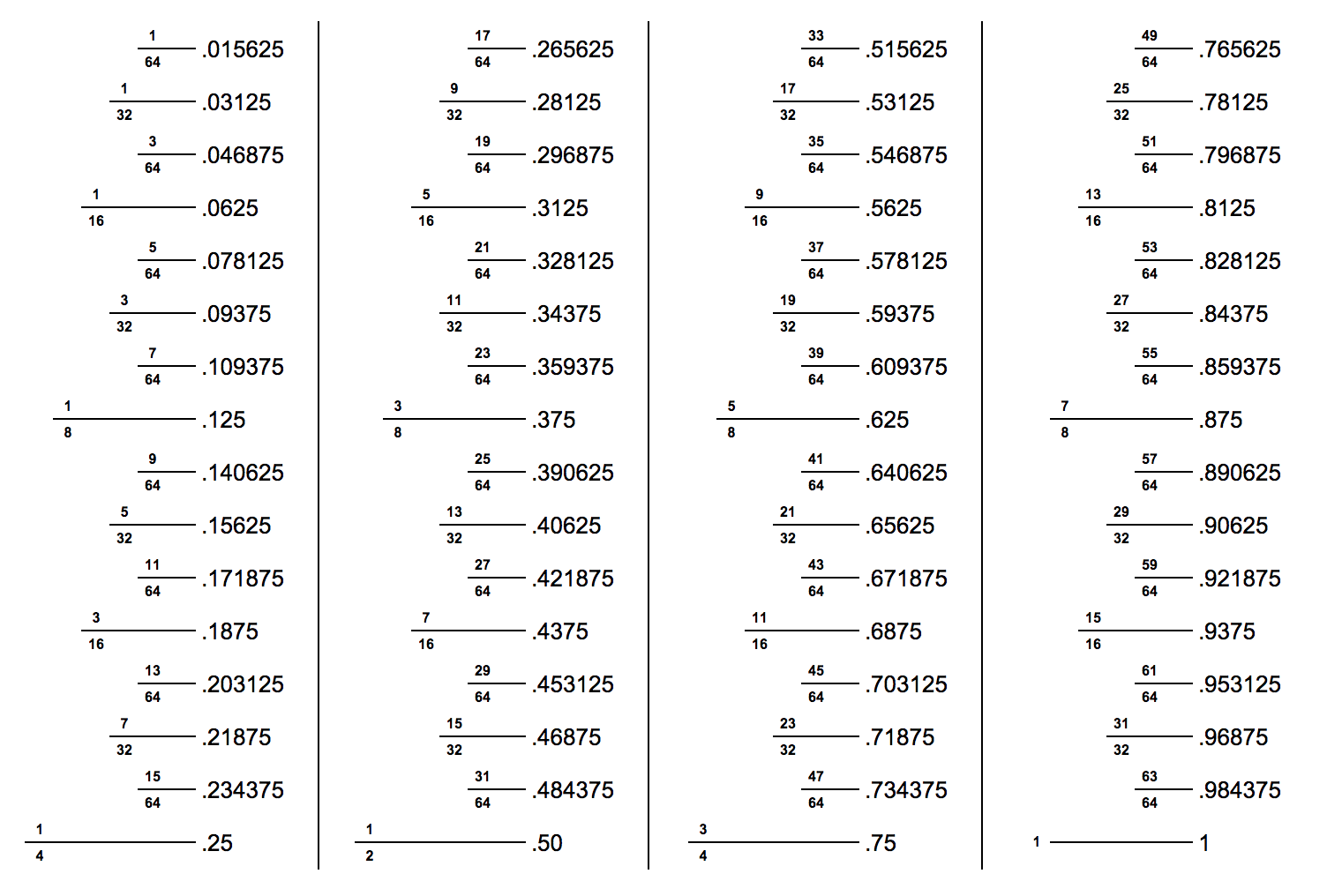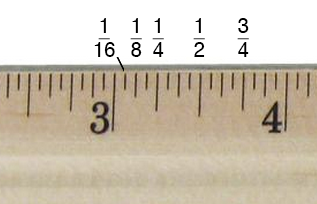# Inch Fraction Calculator – Decimal to Inches

Convert inch fractions to decimal, decimal to inches, inches to metric measurements, metric measurements to inch fractions, and inches to feet using our inches to fraction calculator.## Inch Fraction, Decimal, and Metric Results

5/8"

### Imperial Units

5/8
Inches (fraction)
0.625
Inches
0.052083
Feet

15.875
Millimeters
1.5875
Centimeters
0.015875
Meters

## How to Calculate Inch Fractions

Measurements in inches can be expressed as a decimal or a fraction. When inches are expressed as a fraction, there are some special rules to follow, which are important to know to convert a decimal to inches correctly.

While fractions of an inch can be represented as any standard fraction, the most common fractions of an inch use denominators that are powers of 2, from half inches to up to the 64ths of an inch. That means the fraction denominators are most commonly 2, 4, 8, 16, 32, and 64.

These specific fractions of an inch are referred to as inch fractions.

As a result, if we want to convert a decimal to one of these inch fractions, then know that converting a decimal to an inch fraction is not quite the same as converting a decimal to a regular fraction.

Instead, some minor rounding will occur since it will be necessary to find the nearest fraction with the denominator that is a power of 2, also known as a dyadic fraction or dyadic rational number. Typical inch fractions will look something like 1/64, 1/32, 1/16, 1/8, 1/4, or 1/2.

When you’re working with larger measurements, you can use our feet and inches calculator to add or subtract feet and inch fractions.

### How to Convert Inches to Fraction

To convert decimal inches to an inch fraction, you’ll need to round the decimal portion to the nearest dyadic fractional inch.

First, separate the whole number from the decimal; these are the whole inches. Then, take the remaining portion of the decimal to the right of the decimal point and multiply it by the denominator of the level of precision you need (e.g., 1/16, 1/32, or 1/64).

For instance, to round to the nearest 1/16, take the decimal portion and multiply it by 16. Then, round the result to get the numerator of the inch fraction.

Finally, place the numerator over the level of precision, 16 in this case. Then, put the fractional inches next to the whole inches for the final result.

For example, let’s convert 2.695 inches to a fraction with 1/16″ precision.

Remove the whole number 2 from the decimal to leave us with the decimal 0.695.

Then, multiply 0.695 by 16.

0.695 × 16 = 11.12

Round this to the nearest whole number; in this case, 11.12 can be rounded to 11. Put that in a fraction over 16, so 11/16 is the remaining inch fraction.

Put it all together by placing the whole inches (2) in front of the inch fraction.

2.695 in ≈ 2 11/16

Thus, using this method, you can see that 2.695 decimal inches are approximately equal to 2 11/16 as an inch fraction. Note that we use an “approximately equal” sign instead of an equals sign because the two numbers are not exactly equal.

### Decimal to Inches Chart

Use this decimal to inches chart to easily find the correct fraction for your decimal measurement or to convert inches to fraction. Find decimal equivalents in 164” increments, including 12“, 14“, 18“, 116“, and 132” measurements.

The chart also shows hints on the markings sizes found on a tape measure or ruler.## How to Convert Inch Fractions to Decimal

Converting an inch fraction to decimal inches is as easy as dividing the fraction. Divide the top number (numerator) by the bottom number (denominator) to find the value in inches as a decimal.

You can also use a fraction to decimal calculator or the chart below.

### Inches to Decimal Chart

The inch fraction to decimal and metric chart below shows equivalent length measurements in fraction, decimal, and millimeters up to one inch in 164” increments. You can use this to convert inches to decimal or millimeters.

Inch fractions to the 1/64 of an inch and the equivalent decimal values in inches and metric values in millimeters
Fraction
(inches)
Decimal
(inches)
Metric
(millimeters)
1/64″ 0.015625″ 0.396875 mm
1/32″ 0.03125″ 0.79375 mm
3/64″ 0.046875″ 1.190625 mm
1/16″ 0.0625″ 1.5875 mm
5/64″ 0.078125″ 1.984375 mm
3/32″ 0.09375″ 2.38125 mm
7/64″ 0.109375″ 2.778125 mm
1/8″ 0.125″ 3.175 mm
9/64″ 0.140625″ 3.571875 mm
5/32″ 0.15625″ 3.96875 mm
11/64″ 0.171875″ 4.365625 mm
3/16″ 0.1875″ 4.7625 mm
13/64″ 0.203125″ 5.159375 mm
7/32″ 0.21875″ 5.55625 mm
15/64″ 0.234375″ 5.953125 mm
1/4″ 0.25″ 6.35 mm
17/64″ 0.265625″ 6.746875 mm
9/32″ 0.28125″ 7.14375 mm
19/64″ 0.296875 7.540625 mm
5/16″ 0.3125″ 7.9375 mm
21/64″ 0.328125″ 8.334375 mm
11/32″ 0.34375″ 8.73125 mm
23/64″ 0.359375″ 9.128125 mm
3/8″ 0.375″ 9.525 mm
25/64″ 0.390625″ 9.921875 mm
13/32″ 0.40625″ 10.31875 mm
27/64″ 0.421875″ 10.715625 mm
7/16″ 0.4375″ 11.1125 mm
29/64″ 0.453125″ 11.509375 mm
15/32″ 0.46875″ 11.90625 mm
31/64″ 0.484375″ 12.303125 mm
1/2″ 0.5″ 12.7 mm
33/64″ 0.515625″ 13.096875 mm
17/32″ 0.53125″ 13.49375 mm
35/64″ 0.546875″ 13.890625 mm
9/16″ 0.5625″ 14.2875 mm
37/64″ 0.578125″ 14.684375 mm
19/32″ 0.59375″ 15.08125 mm
39/64″ 0.609375″ 15.478125 mm
5/8″ 0.625″ 15.875 mm
41/64″ 0.640625″ 16.271875 mm
21/32″ 0.65625″ 16.66875 mm
43/64″ 0.671875″ 17.065625 mm
11/16″ 0.6875″ 17.4625 mm
45/64″ 0.703125″ 17.859375 mm
23/32″ 0.71875″ 18.25625 mm
47/64″ 0.734375″ 18.653125 mm
3/4″ 0.75″ 19.05 mm
49/64″ 0.765625″ 19.446875 mm
25/32″ 0.78125″ 19.84375 mm
51/64″ 0.796875″ 20.240625 mm
13/16″ 0.8125″ 20.6375 mm
53/64″ 0.828125″ 21.034375 mm
27/32″ 0.84375″ 21.43125 mm
55/64″ 0.859375″ 21.828125 mm
7/8″ 0.875″ 22.225 mm
57/64″ 0.890625″ 22.621875 mm
29/32″ 0.90625″ 23.01875 mm
59/64″ 0.921875″ 23.415625 mm
15/16″ 0.9375″ 23.8125 mm
61/64″ 0.953125″ 24.209375 mm
31/32″ 0.96875″ 24.60625 mm
63/64″ 0.984375″ 25.003125 mm
1″ 1.0″ 25.4 mm

## How to Convert Inches to Metric Measurements

To convert inch fractions to metric measurements, you need to keep in mind that there are 2.54 centimeters or 25.4 millimeters per inch.

So, to convert an inch fraction to centimeters, you multiply the inches by 2.54, and to convert to millimeters, multiply by 25.4.

cm = in × 2.54
mm = in × 25.4

You can also use a length converter such as an SAE to metric converter.

## How to Find Inch Fractions on a Tape MeasureFinding measurements on a ruler or tape measure can be confusing at first, but once you understand how the marks are laid out, then you’ll see it is quite simple. Notice that the markings between the whole-inch numbers vary in length.

The longest markings will be the quarter-inch markings, i.e., the first marking is 1/4 inch, the second is 1/2 (2/4) inch, and the third is 3/4 inch.

The next longest markings will be the eighth-inch markings, i.e., the first marking is 1/8 inch, the second is 3/8 inch, the third is 5/8 inch, etc.

The smallest markings will be the sixteenth-inch markings, i.e., the first marking is 1/16 inch, the second is 3/16 inch, the third is 5/16 inch, etc.We also have a free printable ruler with fraction measurements marked to help you learn how to find the inch fractions for a measurement. If you need measurements more precise than 64ths of an inch, then you will need to use a more accurate measuring tool such as calipers.

### What is an inch fraction?

An inch fraction is a fraction of an inch with a denominator that is a power of two. For example, 1/2, 3/8, 15/16, 17/32, and 33/64 are all examples of inch fractions.

### How big is one inch?

If you are more familiar with the metric system, one inch is equal to 25.4 millimeters or 2.54 centimeters. If not, a standard paperclip is one inch long, and the diameter of a U.S. quarter is also approximately one inch.

### Do you use decimals or fractions for inches?

You can use either decimals or fractions when representing portions of an inch. Typically we use fractions when the portion of an inch equals one of the common inch fractions in the list above.

### Why does the U.S. use inches?

The U.S. uses inches because it abides by the imperial system of measurements, whereas most of the rest of the world uses the metric system.

The United Kingdom adopted these imperial units in 1826, and since, at the time, it had much influence in the United States, the U.S. adopted them as well. Today, however, the United Kingdom has changed to primarily use the metric system.

### What is the symbol for inches?

Inches are usually represented either with the abbreviation in, or with a double apostrophe, known as a double prime, . For example:

5 inches = 5 in = 5″